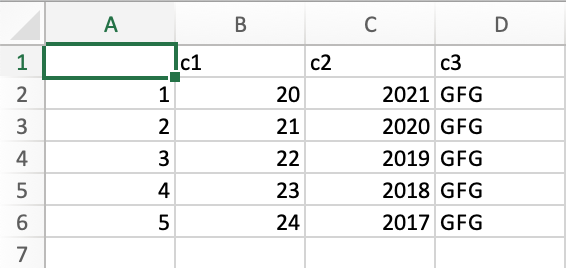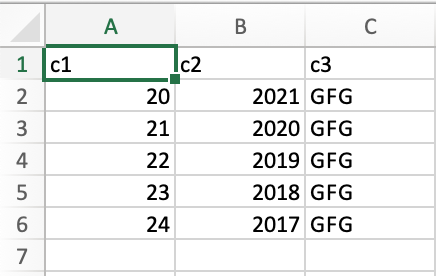# Export CSV File without Row Names in R

• Last Updated : 09 May, 2021

In this article, we will learn how to export CSV files without row names in R Programming Language. In R language we use write.csv() function to create a CSV file from the data.

Syntax: write.csv(df, path)

Parameters:

• df: dataframe object
• path: local path on your system where .csv file will be written/saved.

For this, we have to create a dataframe with the required values and then export values to a dataframe on the provided path. If the file doesn’t exist it firsts create it. If it does, it will be overwritten.

By default, the data is exported with row names. Let us see an example to understand better.

Example:

## R

 `df <- ``data.frame``(c1 = 20:24,``                   ``c2 = ``c``(2021,2020,2019,2018,2017),``                   ``c3 = ``rep``(``"GFG"``, 5))``                    ` `write.csv``(df, ``"dataframe.csv"``)`

Output:Now to export data without row names we simply have to pass row.names=FALSE as an argument in the write.csv() function.

Example:

## R

 `df <- ``data.frame``(c1 = 20:24,``                   ``c2 = ``c``(2021,2020,2019,2018,2017),``                   ``c3 = ``rep``(``"GFG"``, 5))``                    ` `write.csv``(df, ``"dataframe.csv"``, row.names=``FALSE``)`

Output:My Personal Notes arrow_drop_up